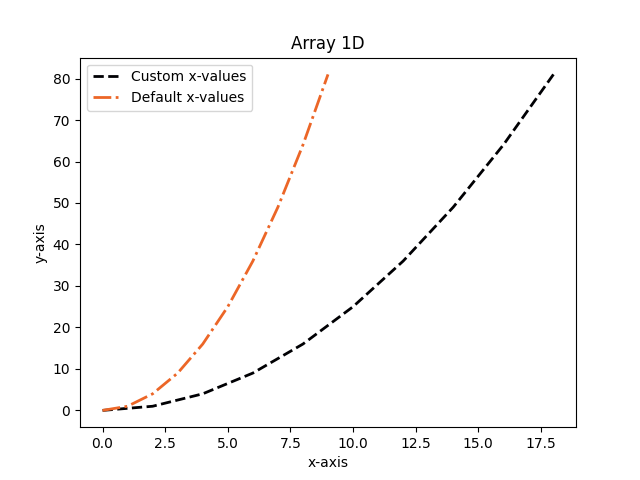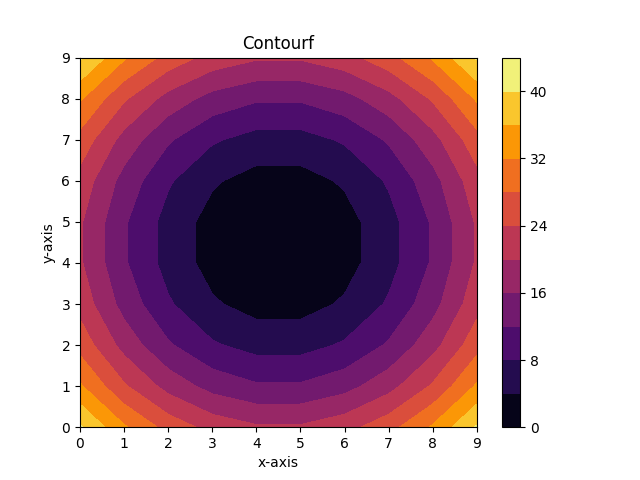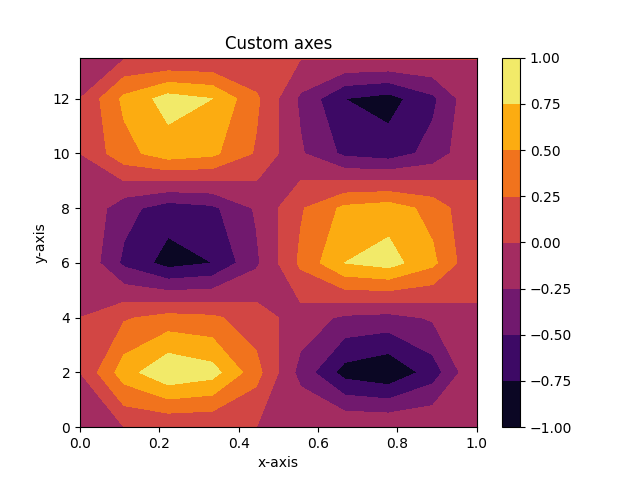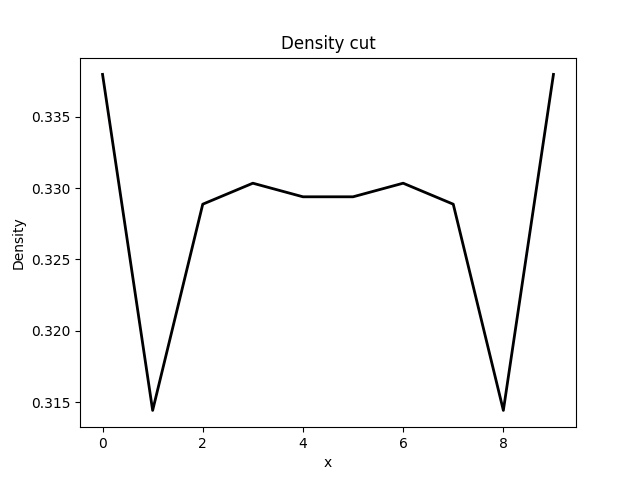TBTK
TBTK::Visualization::MatPlotLib::Plotter Class Reference

Plots data. More...

#include <Plotter.h>

## Public Member Functions

Plotter ()

void setSize (unsigned int width, unsigned int height)

void setBoundsX (double minX, double maxX)

void setBoundsY (double minY, double maxY)

void setBounds (double minX, double maxX, double minY, double maxY)

void setAxes (const std::vector< std::pair< unsigned int, std::vector< double >> > &axes)

void setTitle (const std::string &title, bool overwrite=true)

void setLabelX (const std::string &labelX, bool overwrite=true)

void setLabelY (const std::string &labelY, bool overwrite=true)

void setLabelZ (const std::string &labelZ, bool overwrite=true)

void plot (const Property::Density &density, const Argument &argument="")

void plot (const Index &pattern, const Property::Density &density, const Argument &argument="")

void plot (const Property::DOS &dos, const Argument &argument="")

void plot (const Property::EigenValues &eigenValues, const Argument &argument="black")

void plot (const Property::LDOS &ldos, const Argument &argument="")

void plot (const Index &pattern, const Property::LDOS &ldos, const Argument &argument="")

void plot (const Vector3d &direction, const Property::Magnetization &magnetization, const Argument &argument="")

void plot (const Index &pattern, const Vector3d &direction, const Property::Magnetization &magnetization, const Argument &argument="")

void plot (const Vector3d &direction, const Property::SpinPolarizedLDOS &spinPolarizedLDOS, const Argument &argument="")

void plot (const Index &pattern, const Vector3d &direction, const Property::SpinPolarizedLDOS &spinPolarizedLDOS, const Argument &argument="")

void plot (unsigned int state, const Property::WaveFunctions &waveFunctions, const Argument &argument="")

void plot (const Index &pattern, unsigned int state, const Property::WaveFunctions &waveFunctions, const Argument &argument="")

void plot (const AnnotatedArray< double, double > &data, const Argument &argument="")

void plot (const AnnotatedArray< double, Subindex > &data, const Argument &argument="")

void plot (const Array< double > &data, const Argument &argument="")

void plot (Array< double > x, const Array< double > &y, const Argument &argument="")

void plot (Array< double > x, Array< double > y, const Array< double > &z, const Argument &argument="")

void setPlotMethod3D (const std::string &plotMethod3D)

void setRotation (int elevation, int azimuthal, bool overwrite=true)

void setNumContours (unsigned int numContours)

void clear ()

void show () const

void save (const std::string &filename) const

Ambiguity resolution

These functions resolves otherwise ambiguous function calls.

void plot (const Array< double > &data, const std::initializer_list< std::pair< std::string, std::string > > &argument)

void plot (const std::initializer_list< double > &data, const Argument &argument="")

void plot (const std::initializer_list< double > &x, const Array< double > &y, const Argument &argument="")

void plot (const Array< double > &x, const std::initializer_list< double > &y, const Argument &argument="")

void plot (const std::initializer_list< double > &x, const std::initializer_list< double > &y, const Argument &argument="")

## Detailed Description

Plots data.

The Plotter can plot Properties, Arrays, AnnotatedArrays, and other types that are automatically convertable to these types.

# Matplotlib as backend

The plotter uses matplotlib as backend and therefore requires python with matplotlib to be installed to be possible to use. While the Plotter does not give direct access to matplotlib, it does allow for a subset of plot customization to be done through plot arguments. Each plot function takes an optional list of key-value pairs that will be passed on to the corresponding matplotlib function. For example, it is possible to set the line width and color as follows.

plotter.plot(data, {{"linewidth", "2"}, {"color", "red"}});

It is important to note that the Plotter switches between different matplotlib routines to plot different types of data. The possible key-value pairs depends on which routine is used by the plotter. By default, the Plotter uses the function matplotlib.pyplot.plot for 1D data and matplotlib.pyplot.contourf for 2D data.

# 2D plots

By default 2D data is plotted using matplotlib.pyplot.contourf, but it is also possible to use mpl_toolkit.mplot3d.axes3d.Axes3D.plot_surface. The function that is used can be set using

plotter.setPlotMethod3D("plot_surface");

or

plotter.setPlotMethod3D("contourf");

## Number of contours for contourf

When using contourf, the number of contour levels can be set using

plotter.setNumContours(20);

## Rotation for plot_surface

When using plot_surface, the rotation can be set using

plotter.setRotation(elevation, azimuthal);

where elevation and azimuthal have the type int.

# Title and labels

To set the title and labels, use

plotter.setTitle("My title");
plotter.setLabelX("z-axis");
plotter.setLabelY("y-axis");
plotter.setLabelZ("z-axis");

# Modifying axes

## Bounds

By default axes are rescaled to fit the data. The bounds for a given axis can be changed by calling

plotter.setBoundsX(minX, minY);
plotter.setBoundsY(minY, minY);

or

plotter.setBounds(minX, maxX, minY, maxY);

## Ticks

By default the ticks run from 0 to N-1, where N is the number of data points for the given axis. If the data contans additional information, that allows the tick values to be modified automatically, the Plotter will do so.

For example, EnergyResolvedProperties such as the DOS will have their ticks running between the energy range's lower and upper bound. Similarly, a Property with an Index structure such as {x, y, z}, where x runs from minX to maxX, will have its tick values for the x-axis run from minX to maxX.

It is possible to override this behavior as follows.

plotter.setAxes({
{0, {lowerBound, upperBound}},
{1, {tick0, tick1, tick2}}
});

Here the first line says that the tick values for the first axis should be replaced by uniformly spaced tick values ranging from lowerBound to upperBound. The second line says that the tick values for the second axis should be replaced by tick0, tick1, and tick2. When using the second format, it is important that the number of supplied tick values is the same as the size of the range of the data along that axis. The list of axes supplied to setAxes does not need to be complete and the default behavior will be applied to all axes that are not in the list.

# Properties

Properties can have one of three different formats:

• IndexDescriptor::Format::None
• IndexDescriptor::Format::Ranges
• IndexDescriptor::Format::Custom

The syntax for plotting these differs slightly.

## None and Ranges

Properties on the None and Ranges can be plotted using the syntax

plotter.plot(property, optionalKeyValuePairs);

where property is a Property and optionalKeyValuePairs is an optional list of key-value pairs as described in the matplotlib section above.

## Custom

Properties on the Custom format does not have an explicit structural layout. The Plotter therefore need an additional pattern Index to determine how to plot the data. If, for example, the Property has the Index structure {x, y, z}, it can be plotted for the y=5 plane using

plotter.plot({_a_, 5, _a_}, property, optionalKeyValuePairs);

The number of wildcard falgs *_a_* determines the dimensionallity of the output (plus an additional dimension if the Property has a block structure. All data points satisfying the given pattern will be organized into a grid like structure, and for possible missing data points in this grid, the value will be assumed to be zero.

# Example

#include "TBTK/Array.h"
#include "TBTK/Model.h"
#include "TBTK/PropertyExtractor/Diagonalizer.h"
#include "TBTK/Range.h"
#include "TBTK/Solver/Diagonalizer.h"
#include "TBTK/Streams.h"
#include "TBTK/TBTK.h"
#include "TBTK/Visualization/MatPlotLib/Plotter.h"
using namespace std;
using namespace TBTK;
using namespace Visualization::MatPlotLib;
void plotArray1D(){
const unsigned int SIZE = 10;
Array<double> x({SIZE});
Array<double> y({SIZE});
for(unsigned int n = 0; n < SIZE; n++){
x[{n}] = 2*n;
y[{n}] = n*n;
}
Plotter plotter;
plotter.setTitle("Array 1D");
plotter.setLabelX("x-axis");
plotter.setLabelY("y-axis");
plotter.plot(
x,
y,
{
{"linewidth", "2"},
{"linestyle", "--"},
{"label", "Custom x-values"}
}
);
plotter.plot(
y,
{
{"linewidth", "2"},
{"linestyle", "-."},
{"label", "Default x-values"}
}
);
plotter.save("figures/Array1D.png");
}
void plotDefaultLineStyles(){
const unsigned int SIZE = 100;
Plotter plotter;
plotter.setTitle("Default line styles");
for(unsigned int n = 0; n < 18; n++){
Array<double> y({SIZE});
Range range(0, 2*M_PI, SIZE);
for(unsigned int c = 0; c < range.getResolution(); c++)
y[{c}] = n + sin(range[c]);
plotter.plot(y);
}
plotter.save("figures/DefaultLineStyles.png");
}
void plotArray2D(){
const unsigned int SIZE_X = 10;
const unsigned int SIZE_Y = 10;
Array<double> x({SIZE_X, SIZE_Y});
Array<double> y({SIZE_X, SIZE_Y});
Array<double> z({SIZE_X, SIZE_Y});
for(unsigned int X = 0; X < SIZE_X; X++){
for(unsigned int Y = 0; Y < SIZE_Y; Y++){
x[{X, Y}] = X;
y[{X, Y}] = Y;
z[{X, Y}] = pow(X - 4.5, 2) + pow(Y - 4.5, 2);
}
}
Plotter plotter;
plotter.setNumContours(10);
plotter.setTitle("Contourf");
plotter.setLabelX("x-axis");
plotter.setLabelY("y-axis");
plotter.plot(x, y, z);
plotter.save("figures/Contourf.png");
plotter.clear();
plotter.setPlotMethod3D("plot_surface");
plotter.setTitle("Plot surface");
plotter.setLabelX("x-axis");
plotter.setLabelY("y-axis");
plotter.setLabelZ("z-axis");
plotter.setRotation(30, 60);
plotter.plot(x, y, z);
plotter.save("figures/PlotSurface.png");
}
void plotCustomAxes(){
const unsigned int SIZE_X = 10;
const unsigned int SIZE_Y = 10;
Array<double> x({SIZE_X, SIZE_Y});
Array<double> y({SIZE_X, SIZE_Y});
Array<double> z({SIZE_X, SIZE_Y});
Range kx(0, 2*M_PI, SIZE_X);
Range ky(0, 4*M_PI, SIZE_Y);
for(unsigned int X = 0; X < SIZE_X; X++){
for(unsigned int Y = 0; Y < SIZE_Y; Y++){
x[{X, Y}] = X;
y[{X, Y}] = Y;
z[{X, Y}] = sin(kx[X])*sin(ky[Y]);
}
}
Plotter plotter;
plotter.setTitle("Custom axes");
plotter.setLabelX("x-axis");
plotter.setLabelY("y-axis");
plotter.setAxes({
{0, {0, 1}},
{1, {0, 2, 4, 6, 8, 10, 12, 14, 16, 18}}
});
plotter.setBoundsX(0, 1);
plotter.setBoundsY(0, 13.5);
plotter.plot(x, y, z);
plotter.save("figures/CustomAxes.png");
}
void plotDensity(){
const unsigned int SIZE_X = 10;
const unsigned int SIZE_Y = 10;
double t = 1;
Model model = Models::SquareLattice({SIZE_X, SIZE_Y}, {0, t});
model.construct();
solver.setModel(model);
solver.run();
PropertyExtractor::Diagonalizer propertyExtractor(solver);
= propertyExtractor.calculateDensity({{_a_, _a_}});
Plotter plotter;
plotter.setTitle("Full density");
plotter.plot({_a_, _a_}, density, {{"cmap", "magma"}});
plotter.save("figures/FullDensity.png");
plotter.clear();
plotter.setTitle("Density cut");
plotter.plot({_a_, SIZE_Y/2}, density, {{"linewidth", "2"}});
plotter.save("figures/DensityCut.png");
}
int main(){
plotArray1D();
plotDefaultLineStyles();
plotArray2D();
plotCustomAxes();
plotDensity();
}

## Output## ◆ Plotter()

 TBTK::Visualization::MatPlotLib::Plotter::Plotter ( )
inline

Default constructor.

## ◆ clear()

 void TBTK::Visualization::MatPlotLib::Plotter::clear ( )
inline

Set whether ot not data is plotted on top of old data. Clear the plot and all configuration data.

## ◆ plot() [1/17]

 void TBTK::Visualization::MatPlotLib::Plotter::plot ( const Property::Density & density, const Argument & argument = "" )

Plot point. Plot density on the Property::IndexDescriptor::Format::Ranges format.

Parameters
 density The Property::Density to plot. argument A list of arguments to pass to the underlying matplotlib function. Can either be a single string value or a list such as {{"linewidth", "2"}, {"color", "red"}}.

## ◆ plot() [2/17]

 void TBTK::Visualization::MatPlotLib::Plotter::plot ( const Index & pattern, const Property::Density & density, const Argument & argument = "" )

Plot density on the the Property::IndexDescriptor::Format::Custom format.

Parameters
 pattern An Index pattern that will be used to extract data from the density. For example, if the Index structure of the data contained in the density is {x, y, z}, the pattern {a, 5, a} will result in a plot of the density in the y=5 plane. density The Property::Density to plot. argument A list of arguments to pass to the underlying matplotlib function. Can either be a single string value or a list such as {{"linewidth", "2"}, {"color", "red"}}.

## ◆ plot() [3/17]

 void TBTK::Visualization::MatPlotLib::Plotter::plot ( const Property::DOS & dos, const Argument & argument = "" )

Plot density of states (DOS).

Parameters
 dos The Property::DOS to plot. argument A list of arguments to pass to the underlying matplotlib function. Can either be a single string value or a list such as {{"linewidth", "2"}, {"color", "red"}}.

## ◆ plot() [4/17]

 void TBTK::Visualization::MatPlotLib::Plotter::plot ( const Property::EigenValues & eigenValues, const Argument & argument = "black" )

Plot eigenvalues.

Parameters
 dos The Property::EigenValues to plot. argument A list of arguments to pass to the underlying matplotlib function. Can either be a single string value or a list such as {{"linewidth", "2"}, {"color", "red"}}.

## ◆ plot() [5/17]

 void TBTK::Visualization::MatPlotLib::Plotter::plot ( const Property::LDOS & ldos, const Argument & argument = "" )

Plot local density of states (LDOS) on the IndexDescriptor::Format::Ranges format.

Parameters
 ldos The Property::LDOS to plot. argument A list of arguments to pass to the underlying matplotlib function. Can either be a single string value or a list such as {{"linewidth", "2"}, {"color", "red"}}.

## ◆ plot() [6/17]

 void TBTK::Visualization::MatPlotLib::Plotter::plot ( const Index & pattern, const Property::LDOS & ldos, const Argument & argument = "" )

Plot local density of states (LDOS) on the IndexDescriptor::Format::Custom format.

Parameters
 pattern An Index pattern that will be used to extract data from the LDOS. For example, if the Index structure of the data contained in the LDOS is {x, y, z}, the pattern {5, a, 10} will result in a plot of the LDOS along the line (x, z) = (5, 10). ldos The Property::LDOS to plot. argument A list of arguments to pass to the underlying matplotlib function. Can either be a single string value or a list such as {{"linewidth", "2"}, {"color", "red"}}.

## ◆ plot() [7/17]

 void TBTK::Visualization::MatPlotLib::Plotter::plot ( const Vector3d & direction, const Property::Magnetization & magnetization, const Argument & argument = "" )

Plot magnetization on the IndexDescriptor::Format::Ranges format.

Parameters
 direction The quantization axis to use. magnetization The Property::Magnetization to plot. argument A list of arguments to pass to the underlying matplotlib function. Can either be a single string value or a list such as {{"linewidth", "2"}, {"color", "red"}}.

## ◆ plot() [8/17]

 void TBTK::Visualization::MatPlotLib::Plotter::plot ( const Index & pattern, const Vector3d & direction, const Property::Magnetization & magnetization, const Argument & argument = "" )

Plot magnetization on the IndexDescriptor::Format::Ranges format.

Parameters
 pattern An Index pattern that will be used to extract data from the Magnetization. For example, if the Index structure of the data contained in the Magnetization is {x, y, z}, the pattern {5, a, 10} will result in a plot of the Magnetization along the line (x, z) = (5, 10). direction The quantization axis to use. magnetization The Property::Magnetization to plot. argument A list of arguments to pass to the underlying matplotlib function. Can either be a single string value or a list such as {{"linewidth", "2"}, {"color", "red"}}.

## ◆ plot() [9/17]

 void TBTK::Visualization::MatPlotLib::Plotter::plot ( const Vector3d & direction, const Property::SpinPolarizedLDOS & spinPolarizedLDOS, const Argument & argument = "" )

Plot spin-polarized local density of states (LDOS) on the IndexDescriptor::Format::Ranges format.

Parameters
 direction The quantization axis to use. spinPolarizedLDOS The Property::SpinPolarizedLDOS to plot. argument A list of arguments to pass to the underlying matplotlib function. Can either be a single string value or a list such as {{"linewidth", "2"}, {"color", "red"}}.

## ◆ plot() [10/17]

 void TBTK::Visualization::MatPlotLib::Plotter::plot ( const Index & pattern, const Vector3d & direction, const Property::SpinPolarizedLDOS & spinPolarizedLDOS, const Argument & argument = "" )

Plot spin-polarized local density of states (LDOS) on the IndexDescriptor::Format::Ranges format.

Parameters
 pattern An Index pattern that will be used to extract data from the SpinPolarizedLDOS. For example, if the Index structure of the data contained in the SpinPolarizedLDOS is {x, y, z}, the pattern {5, a, 10} will result in a plot of the SpinPolarizedLDOS along the line (x, z) = (5, 10). direction The quantization axis to use. spinPolarizedLDOS The Property::SpinPolarizedLDOS to plot. argument A list of arguments to pass to the underlying matplotlib function. Can either be a single string value or a list such as {{"linewidth", "2"}, {"color", "red"}}.

## ◆ plot() [11/17]

 void TBTK::Visualization::MatPlotLib::Plotter::plot ( unsigned int state, const Property::WaveFunctions & waveFunctions, const Argument & argument = "" )

Plot wave function on the IndexDescriptor::Format::Ranges format.

Parameters
 state The state number to plot the wave function for. waveFunctions The Property::WaveFunctions to plot from. argument A list of arguments to pass to the underlying matplotlib function. Can either be a single string value or a list such as {{"linewidth", "2"}, {"color", "red"}}.

## ◆ plot() [12/17]

 void TBTK::Visualization::MatPlotLib::Plotter::plot ( const Index & pattern, unsigned int state, const Property::WaveFunctions & waveFunctions, const Argument & argument = "" )

Plot wave function on the IndexDescriptor::Format::Ranges format.

Parameters
 pattern An Index pattern that will be used to extract data from the WaveFunctions. For example, if the Index structure of the data contained in the WaveFunctions is {x, y, z}, the pattern {5, a, 10} will result in a plot of the WaveFunctions along the line (x, z) = (5, 10). state The state number to plot the wave function for. waveFunctions The Property::WaveFunctions to plot. argument A list of arguments to pass to the underlying matplotlib function. Can either be a single string value or a list such as {{"linewidth", "2"}, {"color", "red"}}.

## ◆ plot() [13/17]

 void TBTK::Visualization::MatPlotLib::Plotter::plot ( const AnnotatedArray< double, double > & data, const Argument & argument = "" )

Plot arbitrary data in stored in an AnnotatedArray.

Parameters
 data The data to plot. argument A list of arguments to pass to the underlying matplotlib function. Can either be a single string value or a list such as {{"linewidth", "2"}, {"color", "red"}}.

## ◆ plot() [14/17]

 void TBTK::Visualization::MatPlotLib::Plotter::plot ( const AnnotatedArray< double, Subindex > & data, const Argument & argument = "" )

Plot arbitrary data stored in an AnnotatedArray.

Parameters
 data The data to plot. argument A list of arguments to pass to the underlying matplotlib function. Can either be a single string value or a list such as {{"linewidth", "2"}, {"color", "red"}}.

## ◆ plot() [15/17]

 void TBTK::Visualization::MatPlotLib::Plotter::plot ( const Array< double > & data, const Argument & argument = "" )

Plot arbitrary data stored in an Array.

Parameters
 data The data to plot. argument A list of arguments to pass to the underlying matplotlib function. Can either be a single string value or a list such as {{"linewidth", "2"}, {"color", "red"}}.

## ◆ plot() [16/17]

 void TBTK::Visualization::MatPlotLib::Plotter::plot ( Array< double > x, const Array< double > & y, const Argument & argument = "" )

Plot arbitrary data stored in Arrays.

Parameters
 x The data for the x-axis. y The data for the y-axis. argument A list of arguments to pass to the underlying matplotlib function. Can either be a single string value or a list such as {{"linewidth", "2"}, {"color", "red"}}.

## ◆ plot() [17/17]

 void TBTK::Visualization::MatPlotLib::Plotter::plot ( Array< double > x, Array< double > y, const Array< double > & z, const Argument & argument = "" )

Plot arbitrary data stored in an Array.

Parameters
 x The data for the x-axis. y The data for the y-axis. z The data for the z-axis. argument A list of arguments to pass to the underlying matplotlib function. Can either be a single string value or a list such as {{"linewidth", "2"}, {"color", "red"}}.

## ◆ save()

 void TBTK::Visualization::MatPlotLib::Plotter::save ( const std::string & filename ) const
inline

Save the canvas to file.

Parameters
 filename The file to save the canvas to.

## ◆ setAxes()

 void TBTK::Visualization::MatPlotLib::Plotter::setAxes ( const std::vector< std::pair< unsigned int, std::vector< double >> > & axes )
inline

Set auto scale. Set auto scale. Set auto scale. Set axes values. These axes will override the default axes values. A call can take the following form.

plotter.setAxes({
{axisID0, {lowerBound, upperBound}},
{axisID1, {tick0, tick1, tick2}}
});

Each line corresponds to a separate axis, where the axisID specifies the axis for which to override the default behavior.

If the length of the list corresponding to a given axisID is equal to two, the values will be interpreted as the lower and upper bounds for the plot. If a subsequntly plotted data has N entries for the given axis, element 0 will correspond to the tick value lowerBound, while element N-1 will correspond to upperBound.

If the length of the list corresponding to a given axisID is different from two, the values will be interpreted as tick values. The number of elements in the subsequently plotted data must have the same number of elements for the given axis.

Parameters
 axes List of axes to override the default axes with.

## ◆ setBounds()

 void TBTK::Visualization::MatPlotLib::Plotter::setBounds ( double minX, double maxX, double minY, double maxY )
inline

Set bounds for the x- and y-axes.

Parameters
 minX The minimum value for the x-axis. maxX The maximum value for the x-axis. minY The minimum value for the y-axis. maxY The maximum value for the y-axis.

## ◆ setBoundsX()

 void TBTK::Visualization::MatPlotLib::Plotter::setBoundsX ( double minX, double maxX )
inline

Set bounds for the x-axis.

Parameters
 minX The minimum value for the x-axis. maxX The maximum value for the x-axis.

## ◆ setBoundsY()

 void TBTK::Visualization::MatPlotLib::Plotter::setBoundsY ( double minY, double maxY )
inline

Set bounds for the y-axis.

Parameters
 minY The minimum value for the y-axis. maxY The maximum value for the y-axis.

## ◆ setLabelX()

 void TBTK::Visualization::MatPlotLib::Plotter::setLabelX ( const std::string & labelX, bool overwrite = true )
inline

Set the label for the x-axis.

Parameters
 labelX The label for the x-axis. overwrite If set to false, the label will only be set if it has not already been set.

## ◆ setLabelY()

 void TBTK::Visualization::MatPlotLib::Plotter::setLabelY ( const std::string & labelY, bool overwrite = true )
inline

Set the label for the y-axis.

Parameters
 labelY The label for the y-axis. overwrite If set to false, the label will only be set if it has not already been set.

## ◆ setLabelZ()

 void TBTK::Visualization::MatPlotLib::Plotter::setLabelZ ( const std::string & labelZ, bool overwrite = true )
inline

Set the label for the z-axis.

Parameters
 labelZ The label for the z-axis. overwrite If set to false, the label will only be set if it has not already been set.

## ◆ setNumContours()

 void TBTK::Visualization::MatPlotLib::Plotter::setNumContours ( unsigned int numContours )
inline

Set the number of contours to use when plotting contour plots.

Parameters
 numContours The number of contour levels to use when plotting contour plots.

## ◆ setPlotMethod3D()

 void TBTK::Visualization::MatPlotLib::Plotter::setPlotMethod3D ( const std::string & plotMethod3D )
inline

Plot data with color coded intensity. Plot data with color coded intensity. Set the plot method to use for 3D data.

Parameters
 plotMethod3D The name of the matplotlib function to use for 3D data. The currently supported values are "contourf" and "plot_surface".

## ◆ setRotation()

 void TBTK::Visualization::MatPlotLib::Plotter::setRotation ( int elevation, int azimuthal, bool overwrite = true )
inline

Set rotation angels for 3D plots.

Parameters
 elevation The elevation angle. azimuthal The azimuthal angle. overwrite If set to false, the angles will only be set if they have not already been set.

## ◆ setSize()

 void TBTK::Visualization::MatPlotLib::Plotter::setSize ( unsigned int width, unsigned int height )

Set the canvas size.

Parameters
 width The width of the canvas. height the height of the canvas.

## ◆ setTitle()

 void TBTK::Visualization::MatPlotLib::Plotter::setTitle ( const std::string & title, bool overwrite = true )
inline

Set the plot title.

Parameters
 title The title of the plot. overwrite If set to false, the title will only be set if it has not already been set.

## ◆ show()

 void TBTK::Visualization::MatPlotLib::Plotter::show ( ) const
inline

Show the plot using matplotlibs GUI.

The documentation for this class was generated from the following file:
• /home/kristofer.bjornson/SecondQuantizationCom/TBTK/Lib/include/TBTK/Visualization/MatPlotLib/Plotter.h